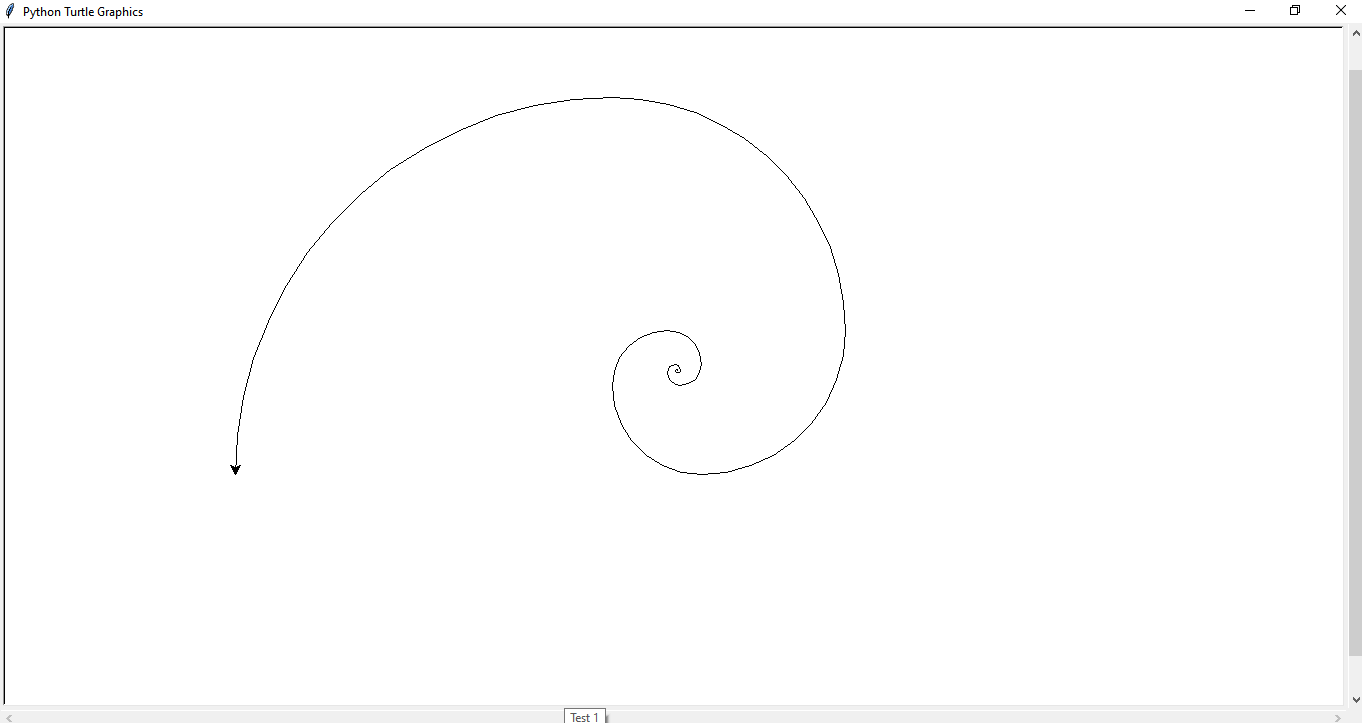Program for Drawing Fibonacci Series Used StructureThe finished program will produce a result like above in a separate screen.

Note :

1. The following program is written on python.

2. You don't need to install turtle module, it is a built-in module.

3.  '#' is used for comments that are ignored by interpreter or compilers

So, Here we go:

#First, we need to import a module( collection of functions )
import turtle

#Then, we need to make a list consisting Fibonacci series (i.e. 0, 1, 1, 2, 3, 5, 8,....) to make a quarter-circle of them

a, b = 0,1
c = 0

fibonacci = []      #List for containing fibonacci numbers we generate by the set of codes below

for i in range(15):        #I have limited the fibonacci sequence to 15 number of terms, so as to                                                #adjust the fibonacci design on the computer screen
fibonacci.append(a)
c = a + b
a = b
b = c

kachuwa = turtle.Turtle()
screen = turtle.Screen()

width = 1300        #adjusting the size of window for showing fibonacci design
height = 750

screen.screensize(width, height)

for number in fibonacci:
kachuwa.circle(number,90)    # .circle() method takes 2 parameter : radius of circle and  to what                                                      #angle the circle is to be drawn(for eg: .circle(5,360) creates a full                                                      # circle  of radius 5 pixels)